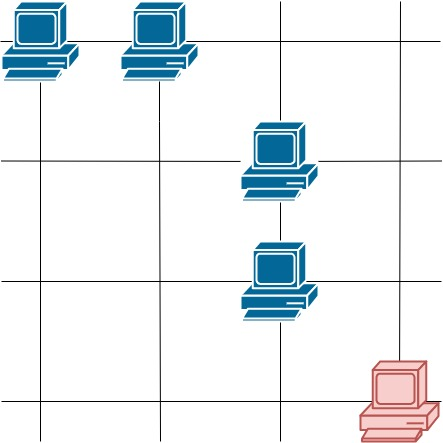# 统计参与通信的服务器

## 1267. 统计参与通信的服务器 (Medium)```输入：grid = [[1,0],[0,1]]```输入：grid = [[1,0],[1,1]]

``````输入：grid = [[1,1,0,0],[0,0,1,0],[0,0,1,0],[0,0,0,1]]

```

• `m == grid.length`
• `n == grid[i].length`
• `1 <= m <= 250`
• `1 <= n <= 250`
• `grid[i][j] == 0 or 1`

[] [数组]